• Stanford机器学习课程笔记——多变量线性回归模型   1. 多变量线性回归模型引入  前面一篇中学习了单变量的线性回归模型，那么自然就会想到多变量线性回归模型，以及非线性模型。这篇我们就学习。  其实...
Stanford机器学习课程笔记——多变量线性回归模型

1. 多变量线性回归模型引入

前面一篇中学习了单变量的线性回归模型，那么自然就会想到多变量线性回归模型，以及非线性模型。这篇我们就学习。
其实，前面的单变量，顾名思义就是样本的特征只有1个，那么多变量就是样本的特征有多个。同样是前面的房屋价格的例子，吴恩达大叔给出了多变量的例子，如下：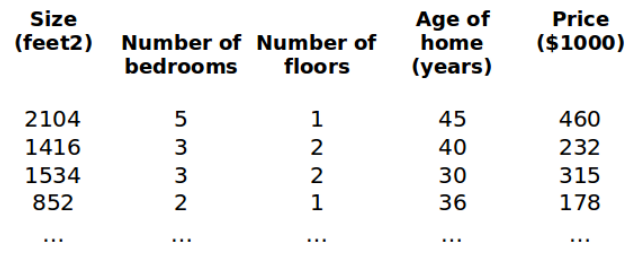这个新例子中，每个样本的特征有4个（房屋面积，卧室个数，楼层，建筑年代），需要许褚的依旧是房屋的价格。
多变量线性回归模型的假设和前面是类似的，只不过theta的个数随着变量个数增加而增加，为：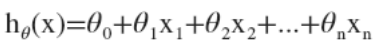。然后我们可以给theta_0配对一个x_0，这样整个形式就一样了。也就是：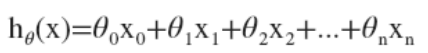，其中我们可以令x_0=1，这样可以转换成常见的向量乘矩阵的形式。也就是：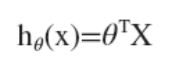。其中的theta是行向量，里面都是线性回归模型中的参数，X是样本矩阵，每一列为一个样本（注意，这里和sklearn中的每一行为一个样本是不一样的）。

有了假设，后面就是代价函数。多变量线性回归模型中的代价函数和单变量的相似，都是样本的误差平方和：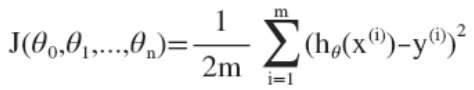只不过其中h_0_x_i中x_i的个数增多了。
同样，我们也可以使用梯度下降法来求解上述目标函数。其粗略的算法步骤如下：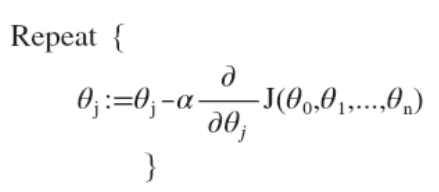其中关键指出在于假设h对所有表示系数theta_i的求导。其实也简单，因为h关于theta的表达式是线性的，那么求导自然就很方便啦，这里我们就直接贴出来吴恩达大叔的原稿：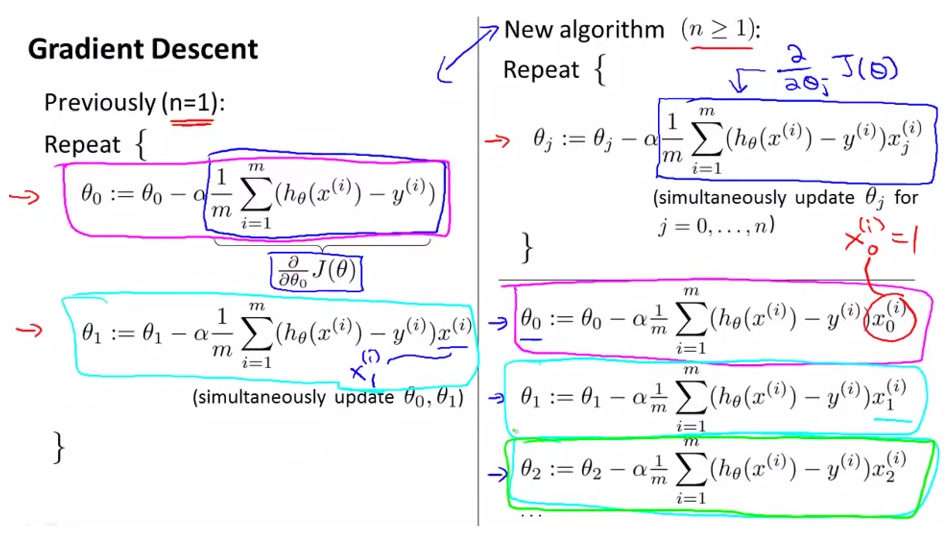这样就得到了更新所有theta时候的下降方向，不过要注意：所有theta_i的更新一定是同时的！

2. 特征缩放

这个特征缩放，我理解类似与特征的归一化normalization. 这是样本多变量情况所特有的。因为样本有多个特征，而每个特征的量纲不完全一样，最大值最小值的取值范围也不同，所以我们需要把它们统一到-1到1之间。
具体而言，对于其中一个特征，我们把所有样本在该特征维度上的值都提取出来，计算均值和标准差。然后利用下面公式归一化：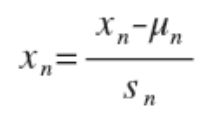其中mu_n是均值，S_n是标准差。这样所有样本在该特征维度上的值均在-1到1之间了。

3. 学习率alpha

这是梯度下降法中的学习率，它决定了在找到下降最快的方向之后，到底下降多少的程度。这是一个度的关系。可以说，梯度下降法这样子万能的求解最优化的选手收到影响的因素就两个，除了初始点的设置之外，还有一个就是它了。
梯度下降法的求解效果收到了学习率大小的影响，如果alpha过小，那么收敛的速度会很慢，求解需要迭代的次数很多；如果alpha很大，那么可能使得更新的时候越过了局部最小值，导致无法收敛，求解失败。（不过这样子看来，还是设置小一些比较稳妥。。）

画出代价函数值关于迭代次数的变化曲线，可以看出alpha设置的是否合理：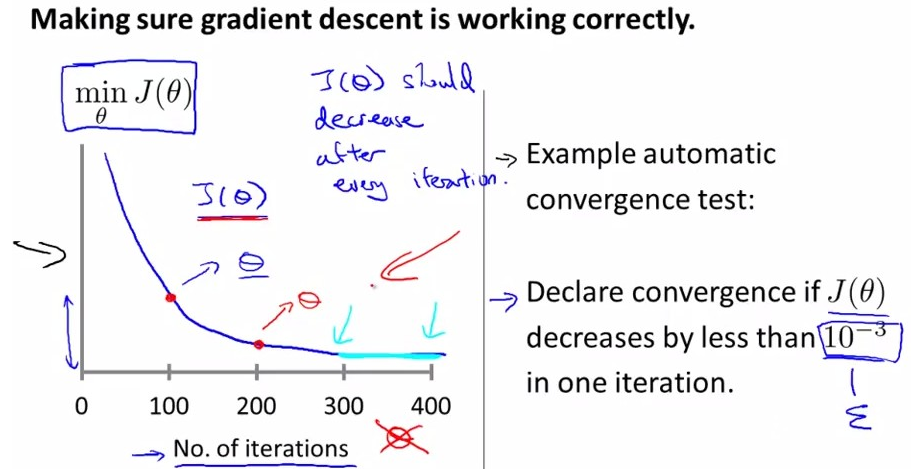而如果alpha设置的不合理，那么代价函数的值可能会逐渐增大，或者在动荡：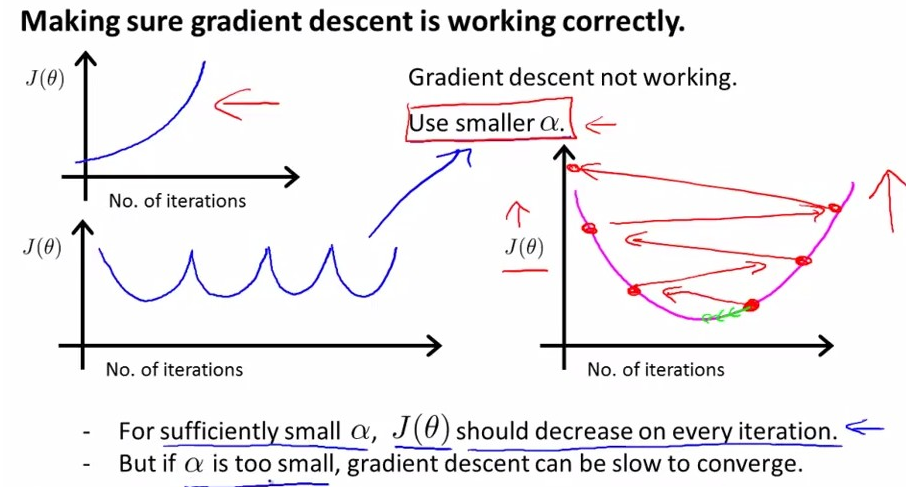上面右图看的比较明显。那么这个学习率到底怎么设置呢？
还是吴恩达大叔给出了建议：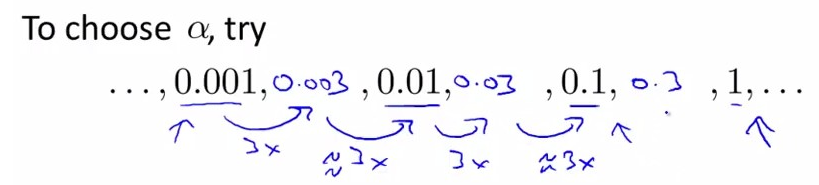看出来了吧，先试一试小的alpha，0.001,如果感觉它太小了，那么再试一试0.01，如果太大了，返回来试一试0.003，这样子一次试下去。。。

4. 非线性回归——多项式回归

开头我们提到了不是所有的数据都满足线性回归的，那么非线性回归又如何呢？这里我们学习一个简单的非线性回归模型，多项式回归，而且它还是可以转化成线性回归的。转换的思路如下：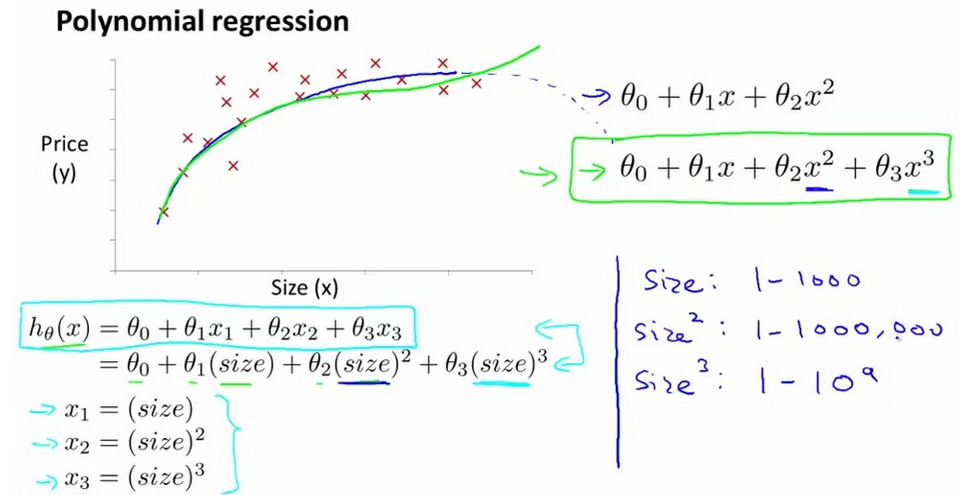也就是说，我们可以把后面的高次项重新用一个新的变量来表示，新的变量只是一次的，这样我们整个模型都是一次的。接下来我们继续可以使用线性回归模型的方法求解。梯度下降法啊，代价函数啊，都是适用的。

5. 正规方程——解析解

课程中说这个是正规方程，刚开始看还不是很理解，后面知道了，其实这里就是解析解。因为我们前面的梯度下降法求得的都是局部最优，不是全局的。这个是解析解，全局的。
说是解析解，是因为回归模型中的代价函数都是可以求导，令导数为零，求得最优解的。那么回到我们刚才说的代价函数形式，我们把假设h的向量乘矩阵的形式带入代价函数中，再求导，即可得：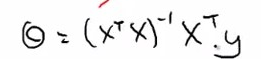其中矩阵X是样本特征矩阵，这个是我们直接就有的，y也是直接有的。也就是说，我们可以把X和y带入上式，就可以一下子求得最优的theta向量了。
但是，我们要注意计算时间复杂度。因为这个求解公司中有矩阵的逆运算，它的时间复杂度是O(n^3)，而且有的时候不是满秩，不可以求逆运算。所以这两个方法（解析解和梯度下降法）各有利弊，总结如下：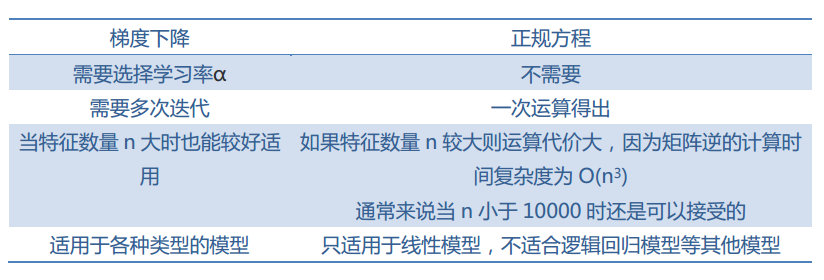其实，遇到不可求逆的情况，分析原因可知：这个因为矩阵不满秩，也就是说代表矩阵X行数的样本特征维数大于代表列数的样本个数，这就是传统的小样本问题。这个时候，我们可以先用PCA降维，把样本的特征维数降低，小于样本的个数，这样就可以了。

已经写到一半的博文突然就没有了，CSDN上面也没有自动保存，晕。。。吐槽一下这个编辑器。。

参考:
http://blog.csdn.net/abcjennifer/article/details/7700772

《Coursera机器学习笔记》
展开全文梯度下降法 学习率 收敛性 小样本问题
• 多变量线性回归模型是监督学习回归问题中较为复杂的模型，因为实际情况时，预测模型不可能仅有一个变量，例如：预测房价时，我们不可能仅考虑房屋面积，还需要考虑所处地、层数、新旧程度等因素。引入此模型之前，先...
由于一些概念在之前的单变量线性回归模型文章已有详细介绍，本篇并不准备再重复一次，如果有不明白的地方可以先看单变量模型，也可以让你更理解本篇。
多变量线性回归模型是监督学习回归问题中较为复杂的模型，因为实际情况时，预测模型不可能仅有一个变量，例如：预测房价时，我们不可能仅考虑房屋面积，还需要考虑所处地、层数、新旧程度等因素。引入此模型之前，先作以下规定。
: 表示训练样本个数
：特征变量的个数
：训练样本第行的输入变量值（向量）
：训练样本第行，第个输入变量的值
：输出变量
：一个训练样本
：第个训练样本
：目标函数，映射

此时我们的目标函数应该是：

如果你的线性代数学的比较好的话，你可以看出它能够表示为两个矩阵的乘积，即  ；其中,，为了方便表示，这里我们规定 = 1，这一点在后面的梯度下降算法中很重要。多变量模型的代价函数和单变量模型类似，给出公式：

此时的代价函数应是一个元函数。我们依旧通过梯度下降算法求代价函数的最小值对应的：
repeat until convergence{

}
求导后我们可以得到：

这个式子对均成立。在每一次迭代时应同时更新，可通过循环的方式，先将值存入一个集合，循环次数设置为，迭代一次完成后再更新所有。
设定好合适的学习率和迭代次数之后，我们就可以得到多变量模型值的全局最优解了。


展开全文• 线性回归分为单变量线性回归多变量线性回归 区别 变量比单变量个特征缩放 代码及注释 import numpy as np import matplotlib.pyplot as plt # 中文、负号 plt.rcParams['font.sans-serif'] = ['SimHei'] plt....
分析
线性回归分为单变量线性回归、多变量线性回归 区别 多变量比单变量多个特征缩放
代码及注释
import numpy as np
import matplotlib.pyplot as plt

# 中文、负号
plt.rcParams['font.sans-serif'] = ['SimHei']
plt.rcParams['axes.unicode_minus'] = False

# 读取数据

# 洗牌
np.random.seed(5)
np.random.permutation(data)

# 提取数据
x,y = data[:,:-1],data[:,-1]

# 数据预处理
def preProcess(x,y):
#特征缩放
x -= np.mean(x,0)
x /= np.std(x,0,ddof=1)

# 数据初始化
x = np.c_[np.ones(len(x)),x]
y = np.c_[y]
return x,y
x,y = preProcess(x,y)

# 切分
train_x,test_x = np.split(x,[int(0.7*len(x))])
train_y,test_y = np.split(y,[int(0.7*len(x))])

# 模型
def model(x,theta):
h = np.dot(x,theta)
return h

# 代价函数
def costFunc(h,y):
e = h - y
j = (1/(2*len(y)))*np.dot(e.T,e)
return j

# 梯度下降函数
m,n = x.shape

# 初始化
theta = np.zeros((n,1))
j_history = np.zeros(max_iter)

for i in range(max_iter):
h = model(x,theta)
j_history[i] = costFunc(h,y)

deltatheta = (1/m)*np.dot(x.T,h-y)
theta -= deltatheta*alpha
return j_history,theta
print(theta)

plt.title('代价函数图像')
plt.plot(j_history)
plt.show()

# 测试值
train_h = model(train_x,theta)
test_h = model(test_x,theta)

# 精度
def score(h,y):
u = np.sum(np.square(h-y))
v = np.sum(np.square(y-np.mean(y)))
return 1-u/v

print('训练集精度:',score(train_h,train_y))
print('测试集精度:',score(test_h,test_y))

plt.title('真实值与测试值对比图')
plt.scatter(train_y,train_y,label='真实值')
plt.scatter(train_y,train_h,label='测试值')
plt.legend()
plt.show()


效果展示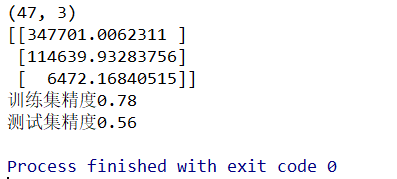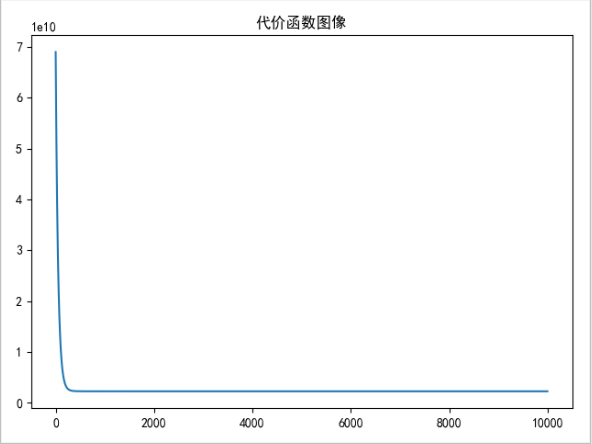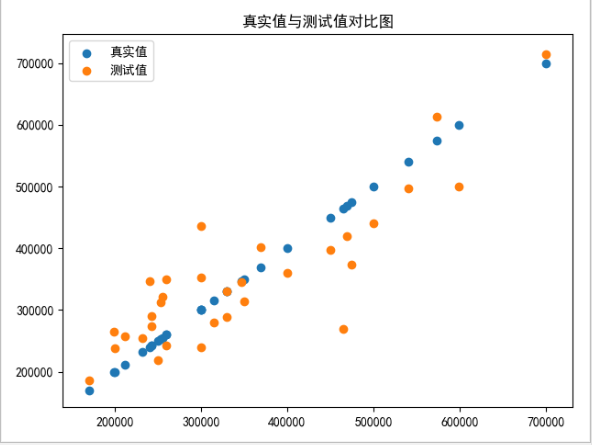展开全文机器学习 有监督学习
• 变量线性回归 import numpy as np import pandas as pd import matplotlib.pyplot as plt path = 'ex1data1.txt' data = pd.read_csv(path, header=None, names=['Population', 'Profit']) data.head()...
机器学习练习 1 - 线性回归
单变量线性回归
import numpy as np
import pandas as pd
import matplotlib.pyplot as plt

path =  'ex1data1.txt'


PopulationProfit06.110117.592015.52779.130228.518613.662037.003211.854045.85986.8233
data.describe()


PopulationProfitcount97.00000097.000000mean8.1598005.839135std3.8698845.510262min5.026900-2.68070025%5.7077001.98690050%6.5894004.56230075%8.5781007.046700max22.20300024.147000
看下数据长什么样子
data.plot(kind='scatter', x='Population', y='Profit', figsize=(12,8))
plt.show()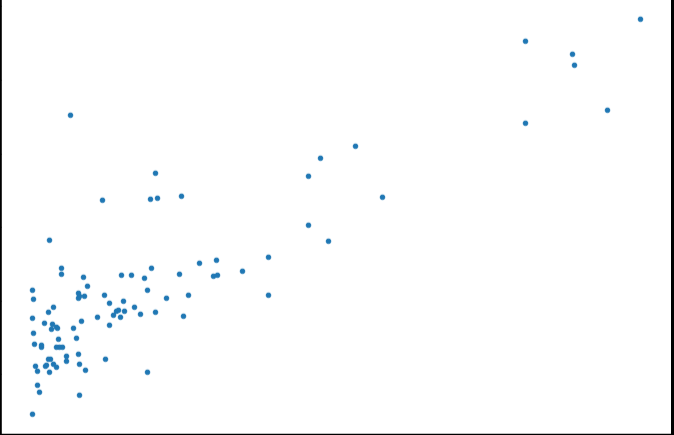现在让我们使用梯度下降来实现线性回归，以最小化成本函数。 以下代码示例中实现的方程在“练习”文件夹中的“ex1.pdf”中有详细说明。
首先，我们将创建一个以参数θ为特征函数的代价函数

J

(

θ

)

=

1

2

m

∑

i

=

1

m

(

h

θ

(

x

(

i

)

)

−

y

(

i

)

)

2

J\left( \theta \right)=\frac{1}{2m}\sum\limits_{i=1}^{m}{{{\left( {{h}_{\theta }}\left( {{x}^{(i)}} \right)-{{y}^{(i)}} \right)}^{2}}}

其中：${{h}{\theta }}\left( x \right)={{\theta }^{T}}X={{\theta }{0}}{{x}{0}}+{{\theta }{1}}{{x}{1}}+{{\theta }{2}}{{x}{2}}+…+{{\theta }{n}}{{x}_{n}}$
def computeCost(X, y, theta):
inner = np.power(((X * theta.T) - y), 2)
return np.sum(inner) / (2 * len(X))

让我们在训练集中添加一列，以便我们可以使用向量化的解决方案来计算代价和梯度。
data.insert(0, 'Ones', 1)

现在我们来做一些变量初始化。
# set X (training data) and y (target variable)
cols = data.shape
X = data.iloc[:,0:cols-1]#X是所有行，去掉最后一列
y = data.iloc[:,cols-1:cols]#X是所有行，最后一列

观察下 X (训练集) and y (目标变量)是否正确.
X.head()#head()是观察前5行


OnesPopulation016.1101115.5277218.5186317.0032415.8598
y.head()


Profit017.592019.1302213.6620311.854046.8233
代价函数是应该是numpy矩阵，所以我们需要转换X和Y，然后才能使用它们。 我们还需要初始化theta。
X = np.matrix(X.values)
y = np.matrix(y.values)
theta = np.matrix(np.array([0,0]))

theta 是一个(1,2)矩阵
theta

matrix([[0, 0]])

看下维度
X.shape, theta.shape, y.shape

((97, 2), (1, 2), (97, 1))

计算代价函数 (theta初始值为0).
computeCost(X, y, theta)

32.072733877455676


θ

j

:

=

θ

j

−

α

∂

∂

θ

j

J

(

θ

)

{{\theta }_{j}}:={{\theta }_{j}}-\alpha \frac{\partial }{\partial {{\theta }_{j}}}J\left( \theta \right)

def gradientDescent(X, y, theta, alpha, iters):
temp = np.matrix(np.zeros(theta.shape))
parameters = int(theta.ravel().shape)
cost = np.zeros(iters)

for i in range(iters):
error = (X * theta.T) - y

for j in range(parameters):
term = np.multiply(error, X[:,j])
temp[0,j] = theta[0,j] - ((alpha / len(X)) * np.sum(term))

theta = temp
cost[i] = computeCost(X, y, theta)

return theta, cost

初始化一些附加变量 - 学习速率α和要执行的迭代次数。
alpha = 0.01
iters = 1000

现在让我们运行梯度下降算法来将我们的参数θ适合于训练集。
g, cost = gradientDescent(X, y, theta, alpha, iters)
g

matrix([[-3.24140214,  1.1272942 ]])

最后，我们可以使用我们拟合的参数计算训练模型的代价函数（误差）。
computeCost(X, y, g)

4.5159555030789118

现在我们来绘制线性模型以及数据，直观地看出它的拟合。
x = np.linspace(data.Population.min(), data.Population.max(), 100)
f = g[0, 0] + (g[0, 1] * x)

fig, ax = plt.subplots(figsize=(12,8))
ax.plot(x, f, 'r', label='Prediction')
ax.scatter(data.Population, data.Profit, label='Traning Data')
ax.legend(loc=2)
ax.set_xlabel('Population')
ax.set_ylabel('Profit')
ax.set_title('Predicted Profit vs. Population Size')
plt.show()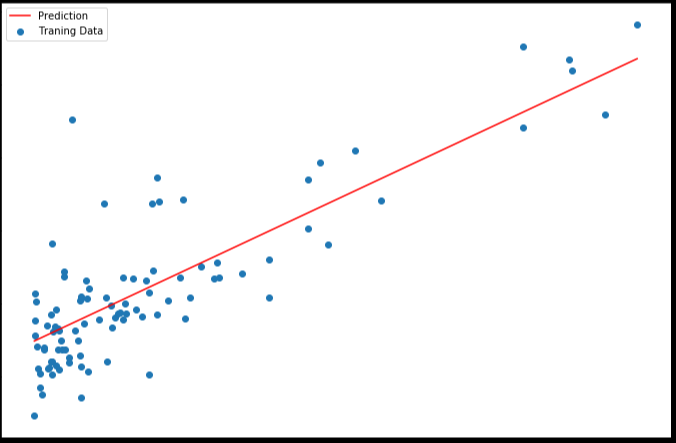由于梯度方程式函数也在每个训练迭代中输出一个代价的向量，所以我们也可以绘制。 请注意，代价总是降低 - 这是凸优化问题的一个例子。
fig, ax = plt.subplots(figsize=(12,8))
ax.plot(np.arange(iters), cost, 'r')
ax.set_xlabel('Iterations')
ax.set_ylabel('Cost')
ax.set_title('Error vs. Training Epoch')
plt.show()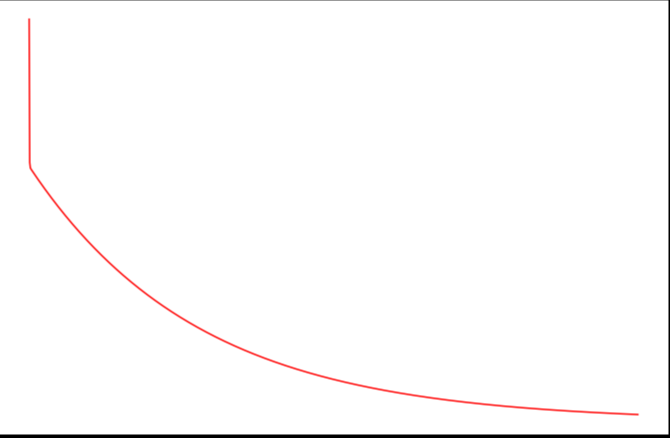多变量线性回归
练习1还包括一个房屋价格数据集，其中有2个变量（房子的大小，卧室的数量）和目标（房子的价格）。 我们使用我们已经应用的技术来分析数据集。
path =  'ex1data2.txt'


SizeBedroomsPrice021043399900116003329900224003369000314162232000430004539900
对于此任务，我们添加了另一个预处理步骤 - 特征归一化。 这个对于pandas来说很简单
data2 = (data2 - data2.mean()) / data2.std()


SizeBedroomsPrice00.130010-0.2236750.4757471-0.504190-0.223675-0.08407420.502476-0.2236750.2286263-0.735723-1.537767-0.86702541.2574761.0904171.595389
现在我们重复第1部分的预处理步骤，并对新数据集运行线性回归程序。
# add ones column
data2.insert(0, 'Ones', 1)

# set X (training data) and y (target variable)
cols = data2.shape
X2 = data2.iloc[:,0:cols-1]
y2 = data2.iloc[:,cols-1:cols]

# convert to matrices and initialize theta
X2 = np.matrix(X2.values)
y2 = np.matrix(y2.values)
theta2 = np.matrix(np.array([0,0,0]))

# perform linear regression on the data set
g2, cost2 = gradientDescent(X2, y2, theta2, alpha, iters)

# get the cost (error) of the model
computeCost(X2, y2, g2)

0.13070336960771892

我们也可以快速查看这一个的训练进程。
fig, ax = plt.subplots(figsize=(12,8))
ax.plot(np.arange(iters), cost2, 'r')
ax.set_xlabel('Iterations')
ax.set_ylabel('Cost')
ax.set_title('Error vs. Training Epoch')
plt.show()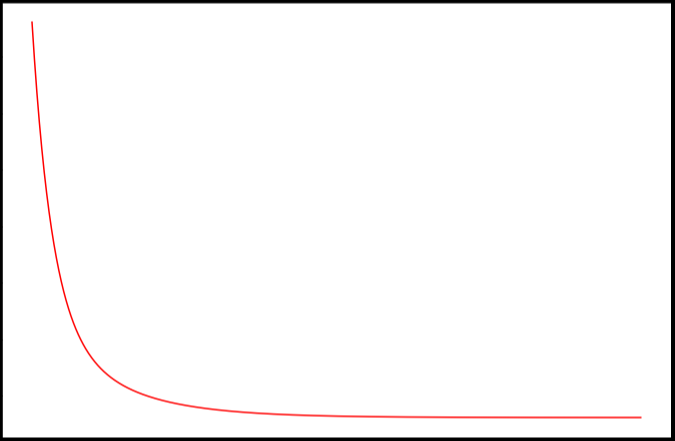我们也可以使用scikit-learn的线性回归函数，而不是从头开始实现这些算法。 我们将scikit-learn的线性回归算法应用于第1部分的数据，并看看它的表现。
from sklearn import linear_model
model = linear_model.LinearRegression()
model.fit(X, y)

LinearRegression(copy_X=True, fit_intercept=True, n_jobs=1, normalize=False)

scikit-learn model的预测表现
x = np.array(X[:, 1].A1)
f = model.predict(X).flatten()

fig, ax = plt.subplots(figsize=(12,8))
ax.plot(x, f, 'r', label='Prediction')
ax.scatter(data.Population, data.Profit, label='Traning Data')
ax.legend(loc=2)
ax.set_xlabel('Population')
ax.set_ylabel('Profit')
ax.set_title('Predicted Profit vs. Population Size')
plt.show()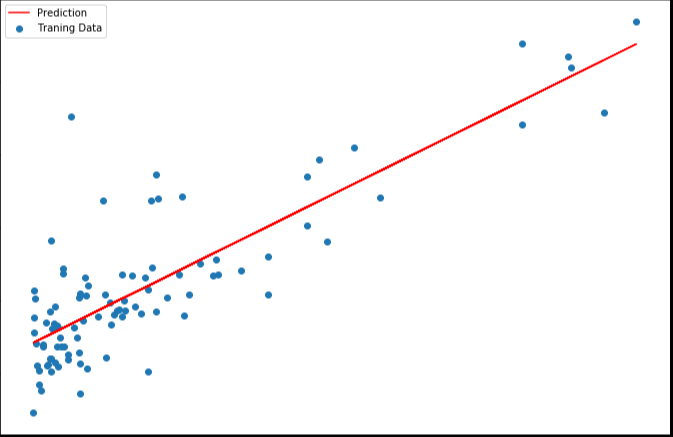4. normal equation（正规方程）
正规方程是通过求解下面的方程来找出使得代价函数最小的参数的：

∂

∂

θ

j

J

(

θ

j

)

=

0

\frac{\partial }{\partial {{\theta }_{j}}}J\left( {{\theta }_{j}} \right)=0

。 假设我们的训练集特征矩阵为 X（包含了

x

0

=

1

{{x}_{0}}=1

）并且我们的训练集结果为向量 y，则利用正规方程解出向量

θ

=

(

X

T

X

)

−

1

X

T

y

\theta ={{\left( {{X}^{T}}X \right)}^{-1}}{{X}^{T}}y

。 上标T代表矩阵转置，上标-1 代表矩阵的逆。设矩阵

A

=

X

T

X

A={{X}^{T}}X

，则：

(

X

T

X

)

−

1

=

A

−

1

{{\left( {{X}^{T}}X \right)}^{-1}}={{A}^{-1}}

梯度下降与正规方程的比较：
梯度下降：需要选择学习率α，需要多次迭代，当特征数量n大时也能较好适用，适用于各种类型的模型
正规方程：不需要选择学习率α，一次计算得出，需要计算

(

X

T

X

)

−

1

{{\left( {{X}^{T}}X \right)}^{-1}}

，如果特征数量n较大则运算代价大，因为矩阵逆的计算时间复杂度为

O

(

n

3

)

O(n3)

，通常来说当

n

n

小于10000 时还是可以接受的，只适用于线性模型，不适合逻辑回归模型等其他模型
# 正规方程
def normalEqn(X, y):
theta = np.linalg.inv(X.T@X)@X.T@y#X.T@X等价于X.T.dot(X)
return theta

final_theta2=normalEqn(X, y)#感觉和批量梯度下降的theta的值有点差距
final_theta2

matrix([[-3.89578088],
[ 1.19303364]])

#梯度下降得到的结果是matrix([[-3.24140214,  1.1272942 ]])

在练习2中，我们将看看分类问题的逻辑回归。


展开全文• 多变量线性回归模型变量线性回归是指输入为多维特征的情况，例如： 在上图中可看出房子的价格price由四个变量(size、number of bedrooms、number of floors 、age of home)决定，为了能够预测给定条件（四个...梯度下降 正规方程 特征正则化 机器学习
• Tensorflow实现梯度下降的单变量线性回归模型，使用到的库：TensorFlow、Numpy、Matplotlib
• 多变量线性回归python实现机器学习前言一、多变量线性回归？3.1 吴恩达多变量线性回归练习3.1.1 版本一3.1.2 版本二3.2 股票预测总结 github地址 前言 机器学习是从人工智能中产生的一个重要学科分支，是实现智能...
• 多变量线性回归模型是单变量线性回归的基础上增加了更的特征，我们生活中需要解决的问题往往都是受多种因素所影响，而引入这些特征后得出的模型适用性更高，以房价预测模型为例。引入房屋尺寸、卧室数量、楼层等...机器学习 有监督学习
• 多变量线性回归1 多变量线性回归应用场景1.1 单变量线性回归案例1.2 多变量线性回归案例2 多元梯度下降法 1 多变量线性回归应用场景 目前为止，我们探讨了单变量/特征的回归模型，现在我们对房价模型增加更的...
• 二、Gradient Descent for Multiple Variables（多变量线性回归的梯度下降） 如何用梯度下降算法来解决特征的线性回归问题？ 1、特征的假设、代价函数和梯度下降函数如下图： 2、将h(x)代入梯度...机器学习
• 文章目录第3章：多变量线性回归3.1 功能 Multiple features3.2 多元梯度下降法 Gradient descent for multiple variables3.3 多元梯度下降法演练I-特征缩放 Gradient descent in practice I:Feature Scaling3.4 ...
• Tensorflow多变量线性回归（房价预测） 。。
• 文章目录单变量线性回归(Linear Regression with One Variable)2.1 模型表示2.2 代价函数2.3 代价函数的直观理解I2.4 代价函数的直观理解II 单变量线性回归(Linear Regression with One Variable) 2.1 模型表示 我们...算法 python 机器学习 人工智能 深度学习
• 在之前的博客中，描述过单变量线性回归(Linear Regression with One Variables)的模型，这次来分享一下多变量线性回归模型(Linear Regression with Multiple Variables)。 我们还是使用之前的问题，对房价的预测...
• 单变量的线性回归模型就不再细说了，这里只记录多变量线性回归中几个需要实现的步骤和函数，其他内容在课程中的讲义和习题中都有详细描述。 特征标准化，对应featureNormalize函数 作用：消除不同特征间的量纲，...机器学习 吴恩达 编程题
• ## 多变量线性回归

千次阅读 2016-12-04 11:03:40
目前为止,我们探讨了单变量/特征的回归模型,现在我们对房价模型增加更的特征, 例如房间数楼层等,构成一个含有变量的模型,模型中的特征为(x1,x2,…,xn)增添更特征后,我们引入一系列新的注释: n 代表特征的...机器学习
•spark 机器学习 sparkMLlib 线性回归
• 模型一般形式 统计模型的一般形式是Y=m(X)+e。其中Y为输出变量、响应变量、因变量、被解释变量；...故模型等式右边是用X组成的函数去描述Y的均值，即模型是在平均的意义下去描述自变量与因变量间的关系...正态分布
• ************************************** 注：本系列博客是博主学习...本系列博客包括线性回归、逻辑回归、神经网络、机器学习的应用和系统设计、支持向量机、聚类、将维、异常检测、推荐系统及大规模机器学习等内容机器学习 正规方程
• R使用lm构建单变量线性回归模型 回归分析是一种应用非常广泛的统计工具，用来建立两个变量之间的关系模型（单变量回归分析）。其中一个变量被称为预测变量（predictor variable），它的值是通过实验收集的。另...
•深度学习
• 继续是机器学习课程的笔记，这节课介绍的是变量的线性回归。多变量线性回归多维特征上节课介绍的是单变量的线性回归，这节课则是进一步介绍变量的线性回归方法。机器学习
• [精选]计量经济学第三版-潘省初-第3章 双变量线性回归模型.pptx
• 回归问题之单变量线性回归：--代价函数--模型表示--梯度下降(梯度下降的线性回归)（一）模型表示我们通过 “学习算法”之 “线性回归算法”，了解监督学习的流程，以之前的房屋交易为例。所用到的参数:m： 训练集中...回归 机器学习
• 四、多变量线性回归(Linear Regression with Multiple Variables) 4.1 多维特征 4.2 变量梯度下降 4.3 梯度下降法实践1-特征缩放 4.4 梯度下降法实践2-学习率 4.5 特征和多项式回归 4.6 正规方程 4.1 多维特征 当...
• 实现线性回归，预测该城市人口对应的利润。 2 数据集描述 现有一个数据集命名为ex1data1.txt，里面包含两个属性，即城市人口和利润。 3 使用工具 Pycharm python3 4 实验步骤 导入数据，并给每列数据命名一个名称...机器学习 python...